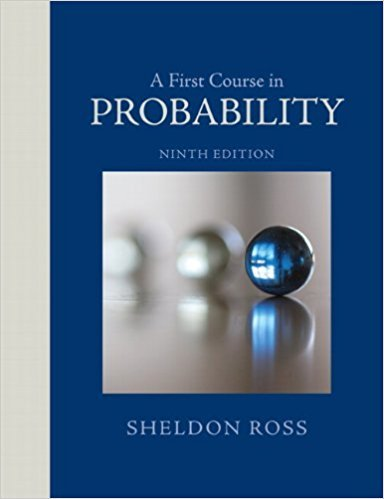×
Get Full Access to A First Course In Probability - 9 Edition - Chapter 4 - Problem 51p
Get Full Access to A First Course In Probability - 9 Edition - Chapter 4 - Problem 51p

×

# The expected number of typographical errors on a page of aISBN: 9780321794772 63

## Solution for problem 51P Chapter 4

A First Course in Probability | 9th Edition

• Textbook Solutions
• 2901 Step-by-step solutions solved by professors and subject experts
• Get 24/7 help from StudySoup virtual teaching assistantsA First Course in Probability | 9th Edition

4 5 1 328 Reviews
21
2
Problem 51P

Problem 51P

The expected number of typographical errors on a page of a certain magazine is .2. What is the probability that the next page you read contains (a) 0 and (b) 2 or more typographical errors? Explain your reasoning!

Step-by-Step Solution:

Solution

Step 1 of 2

Given that the expected no.of typographical errors in a on page of a certain magazine is 0.2

Let X denote the no.of errors in a page

Here X is following the poisson distribution with parameterThe pmf of poisson distribution isa) We have to find the probability that the next page is having zero errors

Now=0.8187

Hence the probability that the next page is having zero errors is 0.8187

Step 2 of 2

##### ISBN: 9780321794772

The full step-by-step solution to problem: 51P from chapter: 4 was answered by , our top Statistics solution expert on 08/11/17, 08:57AM. A First Course in Probability was written by and is associated to the ISBN: 9780321794772. This full solution covers the following key subjects: typographical, errors, page, next, Magazine. This expansive textbook survival guide covers 4 chapters, and 469 solutions. This textbook survival guide was created for the textbook: A First Course in Probability , edition: 9. Since the solution to 51P from 4 chapter was answered, more than 648 students have viewed the full step-by-step answer. The answer to “The expected number of typographical errors on a page of a certain magazine is .2. What is the probability that the next page you read contains (a) 0 and (b) 2 or more typographical errors? Explain your reasoning!” is broken down into a number of easy to follow steps, and 38 words.

Unlock Textbook Solution